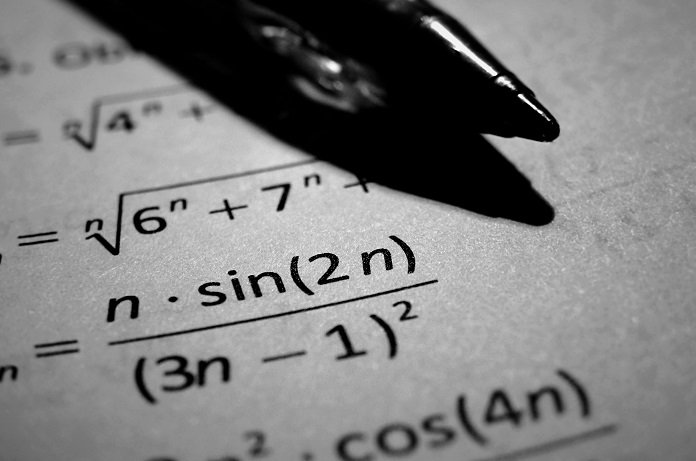Dr. Johnson’s son asks him to help with a math question:
–       Is it true that if I add two odd numbers, I will always get an odd number?
Do you think it is true?

See the answer
Answer:
No, this is not true. Odd numbers are numbers that cannot be divided by 2. For example, 1, 3, 5, 7 and so on.
Pick any two odd numbers, say 3 and 5.
3+5 = 8
8 can be divided by 2, so it is an even, not odd, number.
Facebook Comments